# Chemical Science: Differential Equations

The problem is to find the rate of change of the reactant in a 2nd order chemical reaction which can be modelled by the given by the differential equation,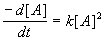, where [A] is the concentration of the reactant at time t in minutes, and k is the reaction constant.

If [ A ] = 0.500 M when t = 0 minutes, and [ A ] = 0.435 M when t = 38.5 minutes, find k .

## Solution:

We need to solve the differential equation:Separate the variables, [ A ] and t , to get: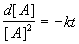Then integrate over the given limits for each variable, to get: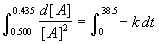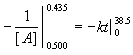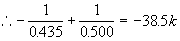∴ And k ≅0.00776Written by Arch McFarlane, June 2003